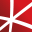# Top 20 NuGet math Packages

.NET native wrapper for the Operations Research Tools project
.NET native wrapper for the Operations Research Tools project
.NET native wrapper for the Operations Research Tools project
The AForge.Math library contains set of math utilities, which are used by other AForge.NET framework's libraries or may be used individually.
Contains a matrix extension library, along with a suite of numerical matrix decomposition methods, numerical optimization algorithms for constrained and unconstrained problems, special functions and other tools for scientific applications. This package is part of the Accord.NET Framework.
The Open Toolkit is set of fast, low-level C# bindings for OpenGL, OpenGL ES and OpenAL. It runs on all major platforms and powers hundreds of apps, games and scientific research. OpenTK provides several utility libraries, including a math/linear algebra package, a windowing system, and input handli...
Math.NET Spatial, providing methods and algorithms for geometry computations in science, engineering and every day use. Supports .Net Framework 4.6.1 or higher and .Net Standard 2.0 or higher on Windows, Linux and Mac.## NMath Premium - Microsoft Chart Controls

Charting for CenterSpace.NMath.Premium types using the Microsoft Chart Controls for .NET, creating a complete data analysis and visualization solution.
Charting for CenterSpace.NMath types using the Microsoft Chart Controls for .NET, creating a complete data analysis and visualization solution.
.NET Standard Library to solve equations expressed as free-form strings with variables, including boolean branching and nested equation trees using forward-only parsing (very fast). Functions include common math, trigonometry and common functions, equation triggers, decimal or double calculation de...
GlmNet is a .NET version of the popular GLM mathematics library.
The Open Toolkit is set of fast, low-level C# bindings for OpenGL, OpenGL ES and OpenAL. It runs on all major platforms and powers hundreds of apps, games and scientific research. OpenTK provides several utility libraries, including a math/linear algebra package, a windowing system, and input handli...
A C# library that supports arbitrary-precision binary and decimal floating-point numbers and rational numbers with arbitrary-precision components, and supports arithmetic with these numbers.
A collection of mathematical function and data structures written in C#. Numerical comparison, vector geometry, polynomial, root solver, statistics, GPS and clustering, and abstract bitmap and draw functions.
This project is a convex hull algorithm and library for 2D, 3D, and higher dimensions. The code can also be used to compute Delaunay triangulations and Voronoi meshes of the input data.
The core of the C# math rendering engine - CSharpMath. Can display beautiful math equations and symbols from the LaTeX format. Needs a front end to function. Currently, front ends exist for iOS, SkiaSharp and Xamarin.Forms.
MyScript Interactive Ink SDK runtime libraries for WPF. Interactive Ink extends digital ink to allow users to more intuitively create, interact with, and share content in digital form. Handwritten text, mathematical equations or even diagrams are interpreted in real-time to be editable v...
The fraction data type consists of two BigInteger values for numerator and denominator. It implements various operations (addition, subtraction, multiplication, division and remainder) with operator overloads.
Extreme Optimization Numerical Libraries for .NET Data Access Library for JSON.## NMath - Standard Library - OSX x64 (alpha)

Foundational classes for financial, engineering, and scientific applications, including complex number classes, general vector and matrix classes, structured sparse matrix classes and factorizations, general sparse matrix classes and factorizations, general matrix decompositions, least squares solut...